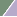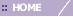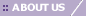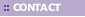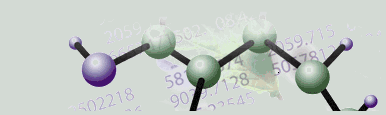# Tutorials/Use Case Scenarios

• This tutorial covers data import and transposition, normalization, renaming experiments, K-Means clustering, matrix tree, centroid, and cluster plots, generating experiment and workflow reports, and exporting images.

• This tutorial covers importing and preprocessing data, renaming datasets, estimating missing values, agglomerative hierarchical clustering, matrix tree plots, color matrix plots, resizing and customizing plots, and generating reports.

• This tutorial covers estimating missing values, normalization, performing Jarvis-Patrick clustering analysis on the datasets from the first two tutorials, and displaying data in a matrix tree plot.

• This tutorial covers importing data, using the table viewer, the summary statistics chart, value removal, filtering, normalization, using Self-Organizing Maps to cluster Leukemia data, visualizing SOM results in a SOM plot and in a cluster plot.

Tutorial 5: Principal Component Analysis (PCA)

• This tutorial demonstrates how to use Principal Component Analysis as a method of extracting more information from data. The tutorial covers data import and displaying PCA results in various plots including: scree, loadings line, color matrix, score (raw and normalized) and 3D score (raw and normalized) plots.

Sample Workflow Using Spotted Array N-Fold Culling With Log Transformation

• This workflow is used for ratio (Cy3/Cy5) data to filter out genes that do not show a large induction or repression in any sample in the dataset, and then to log normalize the data so that inductions and repressions have equal but opposite sign.

• This tutorial demonstrates how to train GeneLinker™ Platinum's artificial neural networks ANNs) to distinguish between sample classes. As an example, data on four similar tumor types is studied. Program features covered include importing variables, the SLAM™ association-mining technology (algorithm and viewer), creating gene lists for filtering, filtering, classification, and classification plots.

• This tutorial demonstrates how to search for a gene to use as an IBIS classifier. One IBIS classifier is produced using Linear Discriminant Analysis (LDA) and a second is produced using Quadratic Discriminant Analysis (QDA). An IBIS Gradient plot is used to analyze the results of the classifier creation.

• This tutorial demonstrates how to use Affymetrix data in GeneLinker™.

• This tutorial demonstrates how to train GeneLinker™ Platinum's committee of SVMs to distinguish between sample classes. As an example, data on two leukemia types is studied. Program features covered include importing variables, creating learners, classification, and classification plots.

•